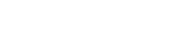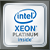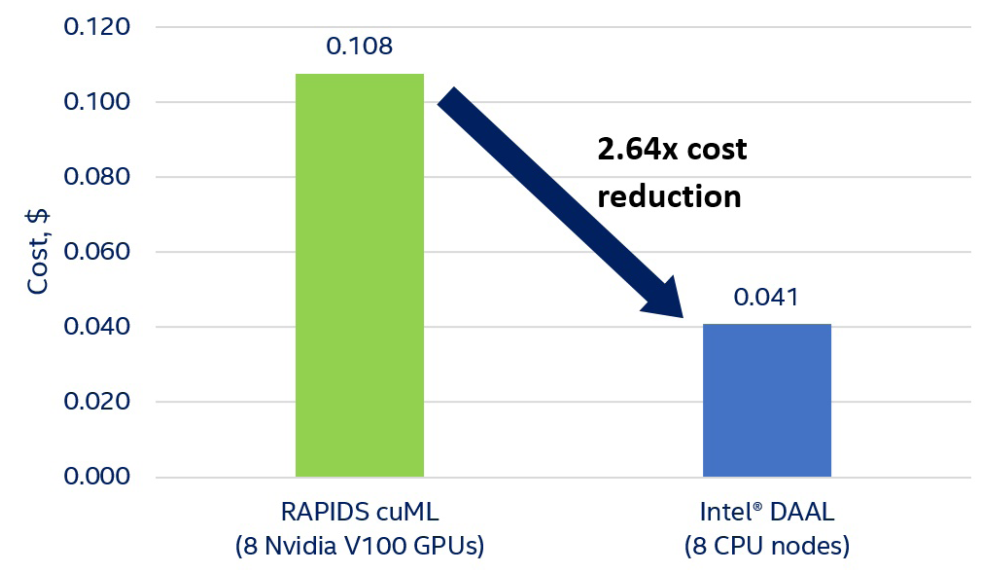Data Science Solution Center

# Accelerate K-Means Clustering

#### Intel’s Hardware and Software for Machine Learning

By Alexander Andreev, Software Engineer and Egor Smirnov, Software Engineering Manager, Intel Corporation

The amount of data humans produce every day is growing exponentially ― and so is the need for high-performance, scalable algorithms to process and extract benefit from all this data. Intel puts great effort into building robust data analytics tools by optimizing not just hardware, but also software. We have built powerful data analytics and machine learning (ML) software [1, 2] that takes advantage of Intel Xeon Scalable processors (which contains Intel Deep Learning Boost technology in the 2nd generation) .

The amount of data humans produce every day is growing exponentially ― and so is the need for high-performance, scalable algorithms to process and extract benefit from all this data. Intel puts great effort into building robust data analytics tools by optimizing not just hardware, but also software. We have built powerful data analytics and machine learning (ML) software [1, 2] that takes advantage of Intel Xeon Scalable processors (which contains Intel Deep Learning Boost technology in the 2nd generation) .

The Intel Distribution for Python (IDP) , an optimized drop-in replacement for the standard Python, includes the scikit-learn ML library. This version of scikit-learn takes advantage of the Intel Data Analytics Acceleration Library (Intel DAAL)  to significantly boost the performance of many classical ML algorithms, including k-means clustering, the subject of this article.

# The K-Means Algorithm

K-means is a simple and powerful ML algorithm to cluster data into similar groups. Its objective is to split a set of N observations into K clusters. This is achieved by minimizing inertia (i.e., the sum of squared Euclidian distances from observations to the cluster centers, or centroids). The algorithm is iterative, with two steps in each iteration:

1. For each observation, compute the distance from it to each centroid, and then reassign each observation to the cluster with the nearest centroid.
2. For each cluster, compute the centroid as the mean of observations assigned to this cluster.

Repeat these steps until the number of iterations exceeds a predefined maximum or the algorithm converges (i.e., the difference between two consecutive inertias is less than a predefined threshold).

Different methods are used to get initial centroids for the first iteration. The algorithm can select random observations as initial centroids or use more complex methods such as k-means++ .

# Intel DAAL versus RAPIDS cuML

To show how Intel DAAL accelerates k-means, we compared it to RAPIDS cuML, which claims to be 90x faster than CPU-based implementations . We also compared cuML with scikit-learn from IDP, which uses Intel DAAL.

We used the following AWS EC2  instances (nodes) to compare performance:

• Multiple (up to 8) c5.24xlarge instances with 2nd Generation Intel Xeon Scalable processor.
• One p3dn.24xlarge instance with eight NVIDIA V100 GPUs.

We used a maximum number of eight c5.24xlarge instances because the cost per hour is approximately equal to one p3dn.24xlarge instance.

The code to generate the synthetic data is shown in the Code Examplessection at the end of this article. We used a data set with 200 million observations, 50 columns, and 10 clusters, which is as much as the V100’s memory can hold. CPU-based systems can typically process much larger data sets. A similar data set with 300 million observations caused a cuML memory error (RMM_ERROR_OUT_OF_MEMORY).

We used the same initialization method in all performance measurements in order to get apples-to-apples comparisons. We also used `float32` data because it gives sufficient accuracy. The K-means algorithm converges to the same result (inertia equals 4×10⁸) for all measured cases. The IDP scikit-learn and Intel DAAL examples are available in the Code Examples section.

Figure 1 and Table 1 show that Intel Xeon processors and Intel DAAL outperform NVIDIA V100 GPUs and RAPIDS cuML. Even one Xeon node is only 40% slower than eight V100 GPUs, which is much better than previous claims of 90x speedup . Also, one Xeon node can hold and process the entire data set, while fewer than eight V100s cannot.

IDP scikit-learn and Intel DAAL running on one Xeon node show approximately the same performance, which isn’t surprising because the scikit-learn library included in IDP takes advantage of Intel DAAL. The slight difference is due to the additional overhead of calling Intel DAAL functions from scikit-learn.

## Faster and Cheaper

We calculated the k-means training cost as the cost of AWS EC2 instances multiplied by the training time for eight Xeon nodes and eight V100 GPUs, respectively .

Figure 2 shows that using Intel DAAL with eight Xeon nodes results in up to 2.64x reduction in k-means training cost.Figure 2. k-means training cost: RAPIDS cuML on 8 GPUs and Intel DAAL on eight CPU nodes.AWS EC2 (N. Virginia) instance prices: c5.24xlarge (Intel DAAL) — \$4.08 per hour(\$32.64 per hour for eight nodes), p3dn.24xlarge (RAPIDS cuML) — \$31.212 per hour.

On AWS EC2, distributed k-means computations are 2.76x faster and 2.64x cheaper with Intel DAAL on eight Xeon nodes than with RAPIDS cuML on eight V100s. Moreover, Xeon-based instances can process much larger data sets. Data that is easily processed on one Xeon node causes “out of memory” errors on multiple V100s. With Intel Optane Memory , capacity increases to 4.5 TB per socket (9 TB per two-socket instance), while an NVIDIA DGX-2 has only 512 GB of GPU memory.

# Code Examples

The best way to install scikit-learn from IDP or daal4py (the Python interface for Intel DAAL)  is by creating a new conda environment:

`conda create -n idp -c intel daal4py scikit-learn pandas`

Data set generation with scikit-learn:

`from sklearn.datasets.samples_generator import make_blobsimport numpy as npx, y = make_blobs(n_samples = 2 * 10**8, n_features = 50,                  centers = 10, cluster_std = 0.2,                  center_box = (-10.0, 10.0), random_state = 777)np.savetxt("kmeans_data.csv", x, fmt = "%f", delimiter = ",")`

Running k-means with scikit-learn from IDP:

`import numpy as npimport pandas as pdimport sklearn as skl# Set config to prevent long finiteness check of input dataskl.set_config(assume_finite = True)# Fast CSV reading with pandastrain_data = pd.read_csv("kmeans_data.csv", dtype = np.float32)alg = skl.cluster.KMeans(n_clusters = 10, init = "random", tol = 0,                         algorithm = "full", max_iter = 50)alg.fit(train_data)`

Running k-means with daal4py:

`import numpy as npimport pandas as pdimport daal4py as d4pd4p.daalinit()data = pd.read_csv("local_kmeans_data.csv", dtype = np.float32)init_alg = d4p.kmeans_init(nClusters = 10, fptype = "float",                           method = "randomDense",                           distributed = True)centroids = init_alg.compute(data).centroidsalg = d4p.kmeans(nClusters = 10, maxIterations = 50,                 fptype = "float", accuracyThreshold = 0,                 assignFlag = False, distributed = True)result = alg.compute(data, centroids)`

# K-Means Training Configuration

CPU configuration: c5.24xlarge AWS EC2 instances; 2nd Generation Intel Xeon Scalable processors, two sockets, 24 cores per socket, HT on, Turbo on, 192 GB RAM (12 slots/16 GB/2933 MHz), BIOS: 1.0 AWS EC2 (ucode: 0x500002c), OS: Ubuntu 18.04.2 LTS.

GPU configuration: p3dn.24xlarge AWS EC2 instance; Intel Xeon Platinum 8175M processor, two sockets, 24 cores per socket, HT on, Turbo on, 768 GB RAM, 8 x NVIDIA Tesla V100-SXM2–32GB GPUs with 32 GB GPU memory each, BIOS 1.0 AWS EC2 (ucode: 0x2000065), OS: Ubuntu 18.04.2 LTS.

Software: Python 3.6, numpy 1.16.4, scikit-learn 0.21.3, daal4py 2019.5, Intel DAAL 2019.5, Intel MPI 2019.5, RAPIDS cuML 0.10, RAPIDS cuDF 0.10, CUDA 10.1, NVIDIA GPU driver 418.87.01, dask 2.6.0.

Algorithm parameters: Single-precision (float32), number of iterations = 50, threshold = 0, random initial centroids.

# References

The original, more detailed version of this article was published in The Parallel Universe (issue 39).